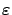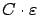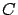Next: Acknowledgments Up: -MDPs: Learning in Varying Previous: Optimal feedback value for

# Conclusions

We have introduced a new model called an-MDP, in which the transition probabilities may change over time as long as the change remains small (-small). The following result--using the generalized MDP framework of [Szepesvári and Littman(1996)]--was proven: if an algorithm converges to the optimal value function in an MDP, then in the corresponding-MDP the asymptotic distance of the optimal value function and its approximation is bounded, and the bound is proportional tounder the same conditions. In other words, an RL algorithm that works well for fixed environments also works well for slightly perturbed environments.

Although in the-MDP model the environment has been allowed to change, it still has to remain within a small neighborhood of some fixed model. This could be extended so that the environment may change (drift) arbitrarily, provided that the drift remains sufficiently slow. It is expected that the algorithm, which is suitable for a fixed environment, may still be able to track a near optimal policy. However, the rigorous proof of this claim is not entirely straightforward; the convergence rate of the algorithm needs to be taken into account.

The theoretical framework of the-MDP model was used to treat a novel RL algorithm, event-learning [Lorincz et al.(2002)]. We have shown that under mild assumptions, event-learning finds a near-optimal value function: if the uncertainty of the underlying controller policy is asymptotically bounded by, then the uncertainty of the resulting value function is at most, whereis a constant depending on the learning problem and the learning parameters. As a consequence of this result, we showed that event-learning augmented with an adapting controller converges to a near-optimal solution. If the policy of the controller is optimal, then the result of event-learning is also optimal. The concepts and theorems of-MDPs, which were designed for event-learning, provide a mathematically attractive framework for RL in perturbed environments as well.

[Givan et al.(2000)] developed a model similar to-MDP s. In their Bounded Parameter MDP (BMDP) model the transition probabilities and the rewards are uncertain in an interval. The value of a state is also an interval between the minimal and maximal possible values. However, they do not give bounds on the size of these value intervals, which can be very large if some transitions are very uncertain. Furthermore, a BMDP is a fixed MDP (but it is uncertain which one), while an-MDP describes an environment that can change over time, even in a slightly non-Markovian way.

Event-learning can be seen as one solution for setting (learning) subgoals, which was originally addressed in modular reinforcement learning [Mahadevan and Connell(1992),Singh(1992),Dayan and Hinton(1993),Kaelbling(1993),Mataric(1997),Kalmár et al.(1998),Dietterich(2000)] and by the formulation of options within a semi-Markov Decision Process framework [Precup and Sutton(1998),Sutton et al.(1998)]. Our formulation has the advantage that a non-Markovian controller can be included. This possibility goes beyond noisy transition probabilities; the robust controller can compensate large changes of some of the parameters. Such large changes may correspond to large changes of the transition probabilities of the original problem and may represent trends of changes. For example, in our pendulum experiment a sudden change of mass was assumed at the very beginning of task execution. This formulation, beyond other features,

• keeps the-MDP property with a particular non-Markovian controller, the SDS controller,
• can deal with uncertain state descriptions, and
• warrants near-optimal performance if the conditions of the theorems are satisfied.
The computer simulations, which were used to illustrate the theory, did not satisfy the time discretization requirements of the theorems. It thus seems that further generalizations could be possible. Additionally, learning the values of state-state transitions is more than optimization of conditioned reflexes or habits, because it concerns desired next states and thus enables direct planning. This issue is under investigation at present.Next: Acknowledgments Up: -MDPs: Learning in Varying Previous: Optimal feedback value for